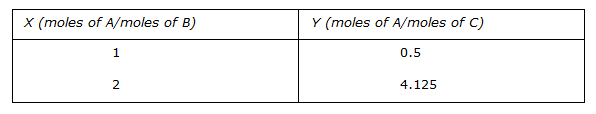# Chemical Engineering - Stoichiometry

### Exercise :: Stoichiometry - Section 5

41.

The density of a gas 'X' is twice that of another gas 'Y'. If the molecular weight of gas 'Y' is 'M'; then the molecular weight of the gas 'X' will be

 A. 2M B. M/2 C. M D. M/4

Explanation:

No answer description available for this question. Let us discuss.

42.

The equilibrium data of component A in the two phases B and C are given below.The estimate of Y for X = 4 by fitting a quadratic expression of a form Y = mX2 for the above data is

 A. 15.5 B. 16 C. 16.5 D. 17

Explanation:

No answer description available for this question. Let us discuss.

43.

Saturated solution of benzene in water is in equilibrium with a mixture of air and vapours of benzene and water at room temperature and pressure. Mole fraction of benzene in liquid is xB and the vapour pressures of benzene and water at these conditions are pvB and pvw respectively. The partial pressure of benzene in air-vapour mixture is

 A. PvB B. xB.PvB C. (Patm - Pvw)xB D. xB.Patm

Explanation:

No answer description available for this question. Let us discuss.

44.

pH value of a solution containing equal concentration of hydroxyl and hydrogen ions will be

 A. 0 B. 10 C. 7 D. 14

Explanation:

No answer description available for this question. Let us discuss.

45.

The percentage ratio of the partial pressure of the vapor to the vapor pressure of the liquid at the existing temperature is

 A. termed as relative saturation. B. not a function of the composition of gas mixture. C. called percentage saturation. D. not a function of the nature of vapor.